# NODE POTENTIAL METHODClick or Tap the Example circuits below to invoke TINACloud then select the Interactive DC mode to Analyze the circuits Online.KIRCHHOFF'S LAWS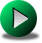MESH AND LOOP CURRENT METHODS

The complete set of Kirchhoff‚Äôs equations can be significantly simplified by the node potential methoddescribed in this chapter. Using this method, Kirchhoff‚Äôs voltage law is satisfied automatically, and we need only write node equations to satisfyKirchhoff‚Äôs current law, too. Satisfying Kirchhoff‚Äôs voltage law is achieved by using node potentials (also called node or nodal voltages)with respect to a particular node called the reference node. In other words, all the voltages in the circuit are relative to the reference node, which is normally considered to have 0 potential. It is easy to see that with these voltage definitions Kirchhoff‚Äôs voltage law is satisfied automatically, since writing loop equations with these potentials leads to identity. Note that for a circuit having N nodes you should write only N - 1 equations. Normally, the node equation for the reference node is left out.

The sum of all currents in the circuit is zero since each current is flowing in and out of a node. Therefore, the Nth node equation is not independent from the previous N-1 equations. If we included all the N equations, we would have an unsolvable system of equations.

The node potential method (also called nodal analysis) is the method best suited to computer applications. Most circuit analysis programs--including TINA--are based on this method.

The steps of the nodal analysis:

1. Pick a reference node with 0 node potential and label each remaining node with V1, V2 or j1, j2and so on.

2. Apply Kirchhoff‚Äôs current law at each node except the reference node. Use Ohm‚Äôs law to express unknown currents from node potentials and voltage source voltages when necessary. For all unknown currents, assume the same reference direction (e.g. pointing out of the node) for each application of Kirchhoff‚Äôs current law.

3. Solve the resulting node equations for the node voltages.

4. Determine any requested current or voltage in the circuit using the node voltages.

Let us illustrate step 2 by writing the node equation for node V1 of the following circuit fragment: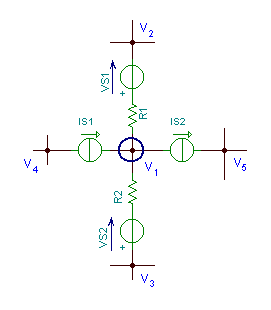First, find the current from node V1 to node V2. We will use Ohm‚Äôs Law at R1. The voltage across R1 is V1 - V2 - VS1

And the current through R1 (and from node V1 to node V2) is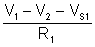Note that this current has a reference direction pointing out of the V1 node. Using the convention for currents pointing out of a node, it should be taken into account in the node equation with a positive sign.

The current expression of the branch between V1 and V3 will be similar, but since VS2 is in the opposite direction from VS1 (which means the potential of the node between VS2 and R2 is V3-VS2), the current is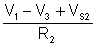Finally, because of the indicated reference direction, IS2 should have a positive sign and IS1 a negative sign in the node equation.

The node equation:Now let‚Äôs see a complete example to demonstrate the use of the node potential method.

Find the voltage V and the currents through the resistors in the circuit below

Click/tap the circuit above to analyze on-line or click this link to Save under Windows

Since we have only two nodes in this circuit, we can reduce the solution to the determination of one unknown quantity.By choosing the lower node as a reference node, the unknown node voltage is the voltage we‚Äôre solving for, V.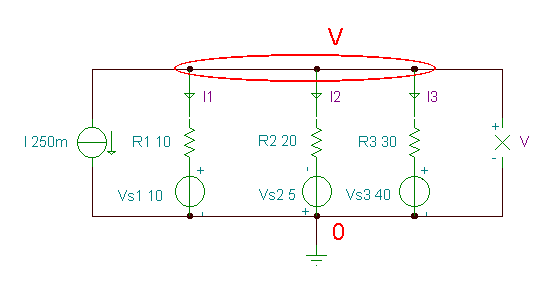Click/tap the circuit above to analyze on-line or click this link to Save under Windows

The nodal equation for the upper node:Numerically: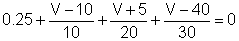Multiply by 30: 7.5+3V ‚Äì 30 + 1.5 V + 7.5.+ V ‚Äì 40 = 0 5.5 V ‚Äì55 = 0

Hence: V = 10 V

 {Solution by TINA's Interpreter} Sys V I+(V-Vs1)/R1+(V+Vs2)/R2+(V-Vs3)/R3=0 end; V=

Now let‚Äôs determine the currents through the resistors. This is easy, since the same currents are used in the nodal equation above.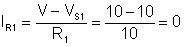{Solution by TINA's Interpreter} {Use node potential method !} Sys V I+(V-Vs1)/R1+(V+Vs2)/R2+(V-Vs3)/R3=0 end; V= {The currents of the resistors} IR1:=(V-Vs1)/R1; IR2:=(V+Vs2)/R2; IR3:=(V-Vs3)/R3; IR1= IR2=[750.0001m] IR3=[-1000m]

We can check the result with TINA by simply turning on TINA‚Äôs DC interactive mode or using the Analysis / DC Analysis / Nodal Voltages command.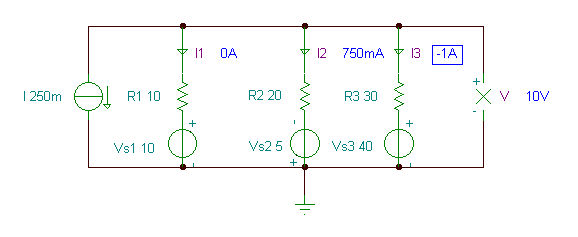Next, let‚Äôs solve the problem which was already used as the last example of the Kirchhoff‚Äôs laws chapter-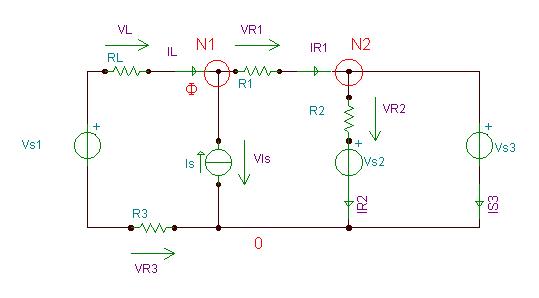Click/tap the circuit above to analyze on-line or click this link to Save under Windows

Find the voltages and currents of each element of the circuit.

Choosing the lower node as a reference node of 0 potential, the nodal voltage of N2 will be equal to VS3, : j2 = therefore we have only one unknown nodal voltage. You may remember that previously, using the full set of Kirchhoff‚Äôs equations, even after some simplifications, we had a linear system of equations of 4 unknowns.

Writing the node equations for node N1, let us denote the nodal voltage of N1 by j1

The simple equation to solve is: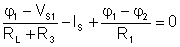Numerically: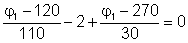Multiply by 330, we get:

3j1-360 ‚Äì 660 + 11j1 ‚Äì 2970 = 0 ¬Æ j1= 285 V

After calculating j1, it is easy to calculate the other quantities in the circuit.

The currents: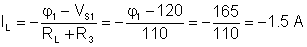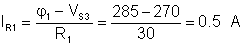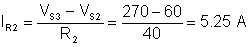IS3 = IR1 ‚Äì IR2 = 0.5 ‚Äì 5.25 = - 4.75 A

And the voltages:

VIs = j1 = 285 V

VR1= (
j1 ‚Äì VS3) = 285 ‚Äì 270 = 15 V

VR2 = (VS3 ‚Äì VS2) = 270 ‚Äì 60 =210 VVL = -(j1-VS1-VR3) = -285 +120 +135 = - 30 V

You may note that with the node potential method you still need some extra calculation to determine the currents and voltages of the circuit. However these calculations are very simple, much simpler than solving linear equations systems for all circuit quantities simultaneously.

We can check the result with TINA by simply turning on TINA‚Äôs DC interactive mode or using Analysis / DC Analysis / Nodal Voltages command.

Let‚Äôs see further examples.

Example 1

Find the current I.

Click/tap the circuit above to analyze on-line or click this link to Save under Windows

In this circuit there are four nodes, but since we have an ideal voltage source that determines the node voltage at its positive pole, we should chose its negative pole as the reference node. Therefore, we really have only two unknown node potentials: j1 and j2 .

Click/tap the circuit above to analyze on-line or click this link to Save under Windows

The equations for the nodes of potentials j1 and j2: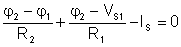Numerically: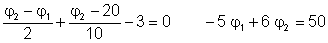so the system of linear equations is: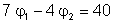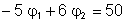To solve this, multiply the first equation by 3 and the second by 2, then add the two equations:

11j1 =220

and hence j1= 20V, j2 = (50 + 5j1) / 6= 25 V

Finally the unknown current: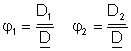The solution of a system of linear equations can be also calculated using Cramer‚Äôs rule.

Let‚Äôs illustrate the use of Cramer‚Äôs rule by solving the system above again..

1. Fill in the matrix of the coefficients of unknowns: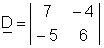2. Calculate the value of the determinant of the D matrix.

| D| = 7*6 ‚Äì (-5)*(-4) = 22

3. Place the values of the right hand side in the column of the coefficients of the unknown variable then calculate the value of the determinant:4.Divide the newly found determinants by the original determinant, to find the following ratios:Hence j1 = 20 V and j2 = 25 V

To check the result with TINA, simply turn on TINA‚Äôs DC interactive mode or use Analysis / DC Analysis / Nodal Voltages command. Note that using the Voltage Pin component of TINA, you can directly show the node potentials assuming that the Ground component is connected to the reference node.

Click/tap the circuit above to analyze on-line or click this link to Save under Windows

 {Solution by TINA's Interpreter} Sys fi1,fi2 (fi1-fi2)/R2+(fi1-VS1)/R3+fi1/R4=0 (fi2-fi1)/R2+(fi2-VS1)/R1-Is=0 end; fi1= fi2= I:=(fi2-VS1)/R1; I=[500m]

# Example 2.

Find the voltage of the resistor R4.

R1 = R3 = 100 ohm, R2 = R4 = 50 ohm, R5 = 20 ohm, R6 = 40 ohm, R7 = 75 ohmClick/tap the circuit above to analyze on-line or click this link to Save under Windows

In this case, it is practical to choose the negative pole of the voltage source VS2 as the reference node because then the positive pole of the VS2 voltage source will have VS2 = 150 node potential. Because of this choice, however, the required V voltage is opposite to the node voltage of the node N4; therefore V4 = - V.

The equations: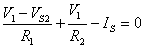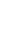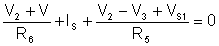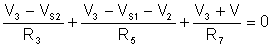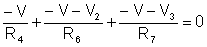We do not present the hand calculations here, since the equations can be easily solved by TINA‚Äôs interpreter.

 {Solution by TINA's Interpreter} {Use node potential method !} Sys V,V1,V2,V3 V1/R2+(V1-Vs2)/R1-Is=0 (V2+V)/R6+(V2-V3+Vs1)/R5+Is=0 (V3+V)/R7+(V3-Vs2)/R3+(V3-Vs1-V2)/R5=0 (-V-V2)/R6-V/R4+(-V-V3)/R7=0 end; V1=[116.6667] V2=[-91.8182] V3=[19.697] V=[34.8485]

To check the result with, TINA simply turn on TINA‚Äôs DC interactive mode or use Analysis / DC Analysis / Nodal Voltages command. Note that we have to place a few voltage pins on the nodes to show the node voltages.

Click/tap the circuit above to analyze on-line or click this link to Save under Windows